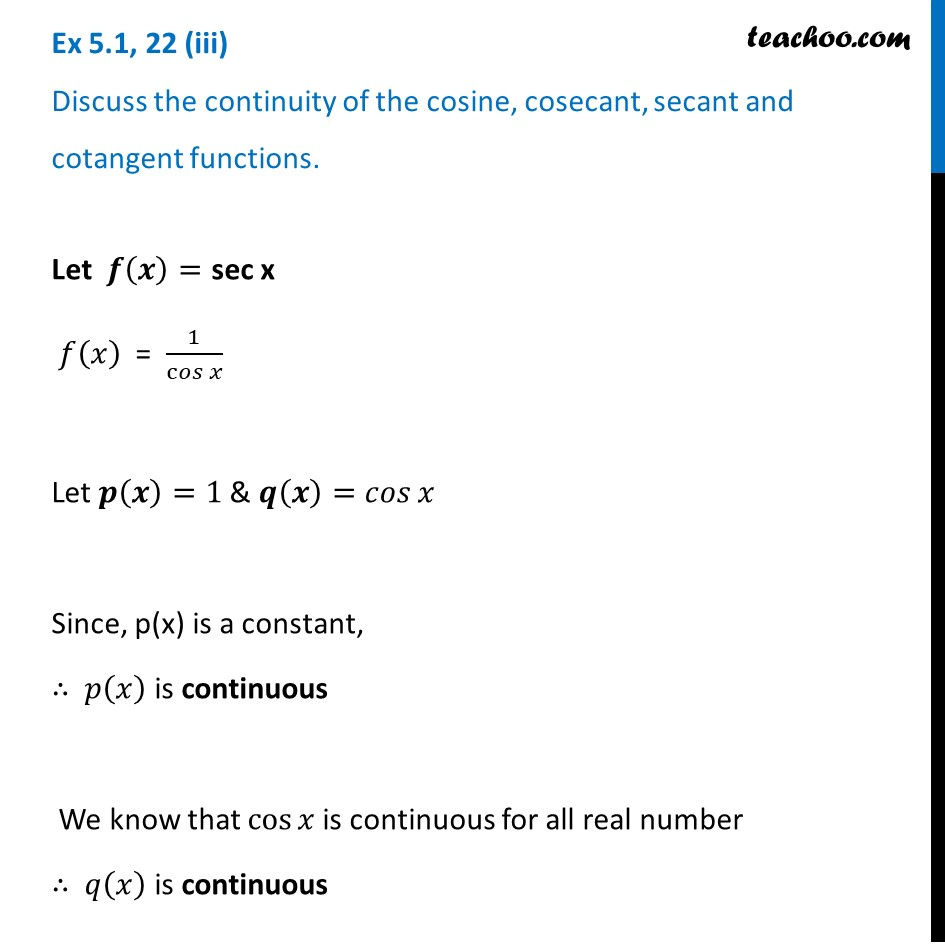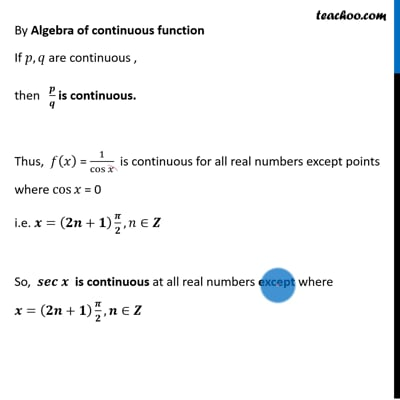Ex 5.1

Chapter 5 Class 12 Continuity and Differentiability
Serial order wiseThis video is only available for Teachoo black users

Introducing your new favourite teacher - Teachoo Black, at only ₹83 per month

### Transcript

Ex 5.1, 22 (iii) Discuss the continuity of the cosine, cosecant, secant and cotangent functions. Let 𝒇(𝒙)="sec x" 𝑓(𝑥) = 1/c𝑜𝑠⁡𝑥 Let 𝒑(𝒙)=1 & 𝒒(𝒙)=𝑐𝑜𝑠⁡𝑥 Since, p(x) is a constant, ∴ 𝑝(𝑥) is continuous We know that cos⁡𝑥 is continuous for all real number ∴ 𝑞(𝑥) is continuous By Algebra of continuous function If 𝑝, 𝑞 are continuous , then 𝒑/𝒒 is continuous. Thus, 𝑓(𝑥) = 1/cos⁡𝑥 is continuous for all real numbers except points where cos⁡𝑥 = 0 i.e. 𝒙=(𝟐𝒏+𝟏) 𝝅/𝟐, 𝑛∈𝒁 So, 𝒔𝒆𝒄 𝒙 is continuous at all real numbers except where 𝒙=(𝟐𝒏+𝟏) 𝝅/𝟐, 𝒏∈𝒁